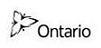# SCIENTIFIC PROGRAMS AND ACTIVITIESAugust 17, 2022THE FIELDS INSTITUTE FOR RESEARCH IN MATHEMATICAL SCIENCES
20th ANNIVERSARY YEAR

 January–June 2013 Thematic Program on Torsors, Nonassociative Algebras and Cohomological Invariants THEMATIC PROGRAM POSTDOCTORAL SEMINARS Stewart LibraryBack to main index

 Upcoming Seminars April 30, 2013 Tuesday 3:30 p.m. Anastasia Stavrova Steinberg groups associated with isotropic reductive groups Past Seminars March 7, 2013 Thursday Uladzimir Yahorau A cohomological proof of Peterson-Kac's theorem on conjugacy of Cartan subalgebras for affine Kac-Moody Lie algebras We say that a subalgebra of a Lie algebra is Cartan if it is ad-diagonalizable and not properly contained in a larger ad-diagonalizable subalgebra. The theorem of Peterson and Kac says that Cartan subalgebras of symmetrizable Kac-Moody Lie algebras are conjugate. We will discuss the proof of this theorem for affine Kac-Moody Lie algebras. Unlike the methods of Peterson and Kac, our approach is entirely cohomological and geometric. This is a joint project with Vladimir Chernousov, Philippe Gille and Arturo Pianzola. March 1, 2013 Zhihua Chang Differential Conformal Superalgebras and Their Twisted Forms In this talk, I will describe the axiomatic definition of a conformal superalgebra introduced by V. G. Kac and its generalization, a differential conformal superalgebra, due to V. G. Kac, M. Lau, and A. Pianzola. This generalization leads to the definition of twisted forms of conformal superalgebras, which can be classified using certain Hˆ1. Finally, I will state our recent results about the automorphism groups and twisted loop conformal superalgebras of the N=1,2,3, small N=4 and large N=4 conformal superalgebras. February 15, 2013 Ting-Yu Lee The embedding of a twisted root datum into a reductive group and the corresponding arithmetic properties First, I would like to describe the relation between the embedding of an étale algebra into a central simple algebra and the embedding of a twisted root datum into a reductive group. In the second part, I will focus on the embedding of a twisted root datum into a reductive group over local fields. In this case, the Tits index determines the existence of the embedding. I would also like to explain the obstruction to the local-global principle for the embedding problem and provide an example when the local-global principle for the embedding problems fail. February 6, 2013 Anastasia Stavrova Isotropic reductive groups over rings Let R be a unital commutative ring. The elementary subgroup E_n(R) of the general linear group GL_n(R) is the subgroup generated by all unipotent elementary matrices in GL_n(R). For any isotropic reductive group scheme G over R, one can define analogs of unipotent elementary matrices, and the respective elementary subgroup E(R) of the group of R-points G(R). When G is a Chevalley group or R is a field, these are the so-called elementary root unipotents in G, parametrized by the roots in the root system of G, that were constructed in the 1950s and 1960s in the work of C. Chevalley, A. Borel, J. Tits, M. Demazure and A. Grothendieck. We will discuss the general case along the lines of the joint papers of the speaker and V. Petrov, and provide some applications to the study of the non-stable K_1-functor, or the Whitehead group, associated to G. January 30, 2013 Jeremy Jacobson On derived Witt groups (Slides) The Witt group of a scheme is a globalization to schemes of the Witt group of a field. It is a part of a cohomology theory for schemes called the derived Witt groups. After an introduction, we recall two problems about the derived Witt groups--the Gersten conjecture and a finite generation question for arithmetic schemes--and then explain recent progress on them. January 23, 2013 Changlong Zhong Formal Group Algebras and Oriented Cohomology of Complete Flags It is known that oriented cohomology of algebraic varieties generalizes the notion of the Chow group and the Grothendieck group, and each oriented cohomology determines a formal group law. On the other hand, the classical characteristic map provides a combinatorial tool to study the Chow group (and the Grothendieck group) of complete flags. In this talk I will introduce the formal group algebras and the characteristic maps introduced by Calmes-Petrov-Zainoulline. Then I will talk about applying it to study the gamma filtration of oriented cohomology of complete flags. This is joint work with J. Malagon-Lopez and K. Zainoulline. January 16, 2013 Wanshun Wong An introduction to Essential Dimension Informally speaking, essential dimension is the smallest number of independent parameters needed to describe an algebraic object. In this talk I will give the definition of essential dimension, and some examples showing how essential dimension is connected to many problems in algebra.